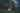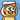# My First Kaggle Competition: Leaf Classification Using Deep Learning Method and with Keras

March 26, 2019# Leaf Classfication

A For CZ4041 Machine Learning Assignment from PT3 in AY2018/2019 Semester 2.

The Kaggle problem is here https://www.kaggle.com/c/leaf-classification/

GitHub repo is here https://github.com/ZhiyueYi/kaggle-leaf-classification

## Import necessary libraries and Define Constants

```import os import csv import pandas as pd import numpy as np import tensorflow as tf from matplotlib import pyplot as plt from keras.models import Sequential from keras.layers import Dense, Activation, Dropout from keras.utils import to_categorical from keras.callbacks import History from keras.preprocessing.text import Tokenizer from sklearn.preprocessing import LabelEncoder, StandardScaler from sklearn.model_selection import StratifiedShuffleSplit from collections import Counter``` `Using TensorFlow backend.`

Histories are to record model losses in every epoch

```history1 = History() history2 = History() history3 = History() LABEL_PATH = 'data/' TRAIN_FILE_NAME = 'train.csv' TEST_FILE_NAME = 'test.csv'```

Load features and labels from the train csv file

y is extracted from the `species` column and converted from text string to numeric values as classes

x contains features in the remaining columns except `id` columns. `StandardScaler` is used to transform the data so that its distribution will have a `mean = 0` and standard `deviation = 1`. It is to standardize the scale of the data for ease of computation and remain the features unaffected.

```train_data_frame = pd.read_csv(LABEL_PATH + TRAIN_FILE_NAME) train_data_frame = train_data_frame.drop(['id'], axis=1) y = train_data_frame.pop('species') classes = np.unique(y) y = to_categorical(LabelEncoder().fit(y).transform(y)) x = StandardScaler().fit(train_data_frame).transform(train_data_frame)```

Use `StratifiedShuffleSplit` to randomly split the data set into training data and validation data.

```sss = StratifiedShuffleSplit(n_splits=10, test_size=0.2,random_state=12345) train_index, validation_index = next(iter(sss.split(x, y))) train_x, validate_x = x[train_index], x[validation_index] train_y, validate_y = y[train_index], y[validation_index] print("train_x dimention: ",train_x.shape) print("validate_x dimention: ",validate_x.shape)``` ```train_x dimention: (792, 192) validate_x dimention: (198, 192)```

Get the number of classes for later computation

`no_of_classes = len(np.unique(train_y, axis=0))`

## Build model

Use ensemble learning method to predict the value.

Ensemble learning refers to training multiple models with the same set of training data set and validation data set. With multiple sets of models, a pool of predicted values can be generated. We can pick the most possible predicted value from the pool to achieve the best accuracy.

```model1 = Sequential() model1.add(Dense(250, activation='relu', input_dim = train_x.shape)) model1.add(Dropout(0.2)) model1.add(Dense(150, activation='relu')) model1.add(Dropout(0.4)) model1.add(Dense(no_of_classes, activation=tf.nn.softmax))``` ```model2 = Sequential() model2.add(Dense(1000, activation='tanh', input_dim = train_x.shape)) model2.add(Dense(1000, activation='relu')) model2.add(Dense(1000, activation='relu')) model2.add(Dense(no_of_classes, activation=tf.nn.softmax))``` ```model3 = Sequential() model3.add(Dense(500, activation='relu', input_dim = train_x.shape)) model3.add(Dropout(0.4)) model3.add(Dense(500, activation='relu')) model3.add(Dropout(0.2)) model3.add(Dense(no_of_classes, activation=tf.nn.softmax))``` `model1.summary()` ```_________________________________________________________________ Layer (type) Output Shape Param # ================================================================= dense_1 (Dense) (None, 250) 48250 _________________________________________________________________ dropout_1 (Dropout) (None, 250) 0 _________________________________________________________________ dense_2 (Dense) (None, 150) 37650 _________________________________________________________________ dropout_2 (Dropout) (None, 150) 0 _________________________________________________________________ dense_3 (Dense) (None, 99) 14949 ================================================================= Total params: 100,849 Trainable params: 100,849 Non-trainable params: 0 _________________________________________________________________``` `model2.summary()` ```_________________________________________________________________ Layer (type) Output Shape Param # ================================================================= dense_4 (Dense) (None, 1000) 193000 _________________________________________________________________ dense_5 (Dense) (None, 1000) 1001000 _________________________________________________________________ dense_6 (Dense) (None, 1000) 1001000 _________________________________________________________________ dense_7 (Dense) (None, 99) 99099 ================================================================= Total params: 2,294,099 Trainable params: 2,294,099 Non-trainable params: 0 _________________________________________________________________``` `model3.summary()` ```_________________________________________________________________ Layer (type) Output Shape Param # ================================================================= dense_8 (Dense) (None, 500) 96500 _________________________________________________________________ dropout_3 (Dropout) (None, 500) 0 _________________________________________________________________ dense_9 (Dense) (None, 500) 250500 _________________________________________________________________ dropout_4 (Dropout) (None, 500) 0 _________________________________________________________________ dense_10 (Dense) (None, 99) 49599 ================================================================= Total params: 396,599 Trainable params: 396,599 Non-trainable params: 0 _________________________________________________________________```

## Compile and fit the model

At this stage, the data is pumped into the model and Keras will help to run iterations to reduce the loss as much as possible.

```model1.compile(loss='categorical_crossentropy', optimizer='adam', metrics=['accuracy']) model1.fit(train_x, train_y, epochs = 50, verbose=0, validation_data=(validate_x, validate_y), callbacks=[history1])``` `<keras.callbacks.History at 0x171c05a8240>` ```model2.compile(loss='categorical_crossentropy', optimizer='adam', metrics=['accuracy']) model2.fit(train_x, train_y, epochs = 10, verbose=0, validation_data=(validate_x, validate_y), callbacks=[history2])``` `<keras.callbacks.History at 0x171c0e12438>` ```model3.compile(loss='categorical_crossentropy', optimizer='adam', metrics=['accuracy']) model3.fit(train_x, train_y, epochs = 20, verbose=0, validation_data=(validate_x, validate_y), callbacks=[history3])``` `<keras.callbacks.History at 0x171c1450908>`

## Save Models

Trained model information is saved for future use.

```model1.save('models/model_1_0.29073.h5') model2.save('models/model_2_0.29073.h5') model3.save('models/model_3_0.29073.h5')```

## Test

Repeat the same data pre-processing procedures on the test dataset

```test_data_frame = pd.read_csv(LABEL_PATH + TEST_FILE_NAME) index = test_data_frame.pop('id') test_x = StandardScaler().fit(test_data_frame).transform(test_data_frame) #test_x = test_data_frame.get_values()```

Separately use the 3 models to predict the results based on the test X values.

```test_y_1 = model1.predict_classes(test_x) test_y_2 = model2.predict_classes(test_x) test_y_3 = model3.predict_classes(test_x)```

A summarised `test_y` object is generated and sorted by `loss` in ascending order.

```test_y = [ { 'name': 'test_y_1', 'predict': test_y_1, 'loss': history1.history['loss'][-1] }, { 'name': 'test_y_2', 'predict': test_y_2, 'loss': history2.history['loss'][-1] }, { 'name': 'test_y_3', 'predict': test_y_3, 'loss': history3.history['loss'][-1] }, ] test_y = sorted(test_y, key=lambda k: k['loss'])```

`data_grid` is used to store the generated the file format which Kaggle competition will accept.

Then iterate every predicted data and compare the results in the 3 models.

If 2 or more models predict the same class, it will be the actual predicted class.

If 3 models all predict different classes, then the value of the model with the least loss will be athe actual predicted class.

```data_grid = np.zeros((len(test_y_1), len(classes))) for i in range(len(test_y_1)): count = {} for test in test_y: if test['predict'][i] not in count: count[test['predict'][i]] = 1 else: count[test['predict'][i]] += 1 result = Counter(count) predicted = result.most_common(1) data_grid[i][predicted] = 1```

Use `pd.DataFrame` to generate CSV format variable.

`prediction = pd.DataFrame(data_grid, index = index, columns = classes)`

Lastly, write the variable into the CSV file for submission.

```with open('submission.csv','w') as file: file.write(prediction.to_csv())```

Under the same directory, run `kaggle competitions submit -c leaf-classification -f submission.csv -m "Message"` command to submit the CSV file to Kaggle.

# Thanks…

Feature image credits to https://www.pexels.com/photo/brown-dried-leaf-767956/Written by Yi Zhiyue
A Software Engineer · 山不在高，有仙则灵